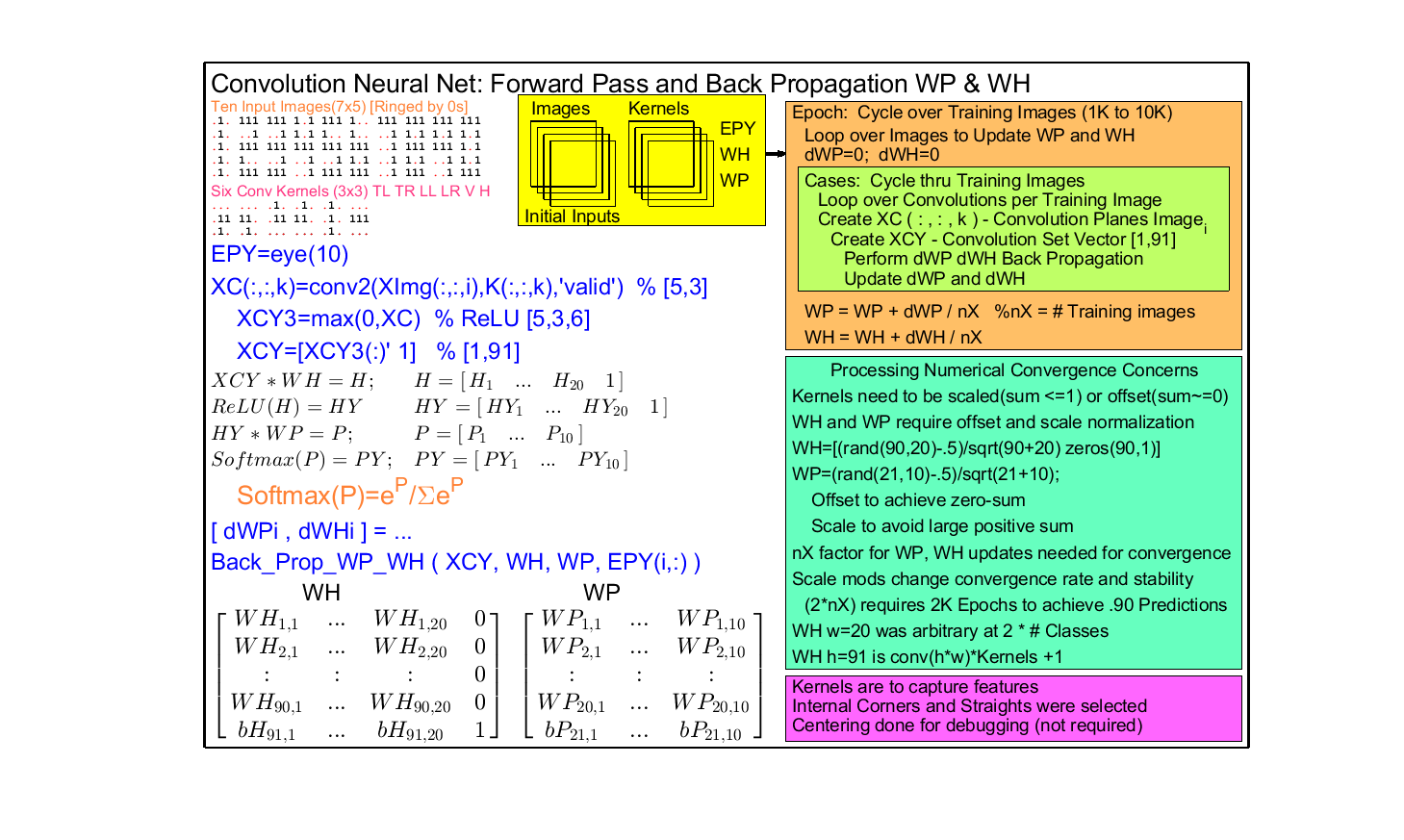# Problem 58926. Neural Net: Convolutional NN Matrices Determination for LED Digit images

This challenge is to return final WH and WP matrices, given XImgset, WH, WP, K, EPY using convolution(Ximg,K), ReLU on the hidden layer and Softmax on the output layer. Training images are LED binary matrices of digits 1:9 and 0. A fun extension is to add LED variants and see if trained WH/WP give expected result.
[WP,WH]=ConvNN_Process(XImgset,K,WH,WP,EPY)The matlab Latex code for making the CNN Processing chart included in template.

### Solution Stats

100.0% Correct | 0.0% Incorrect
Last Solution submitted on Sep 26, 2023

### Community Treasure Hunt

Find the treasures in MATLAB Central and discover how the community can help you!

Start Hunting!